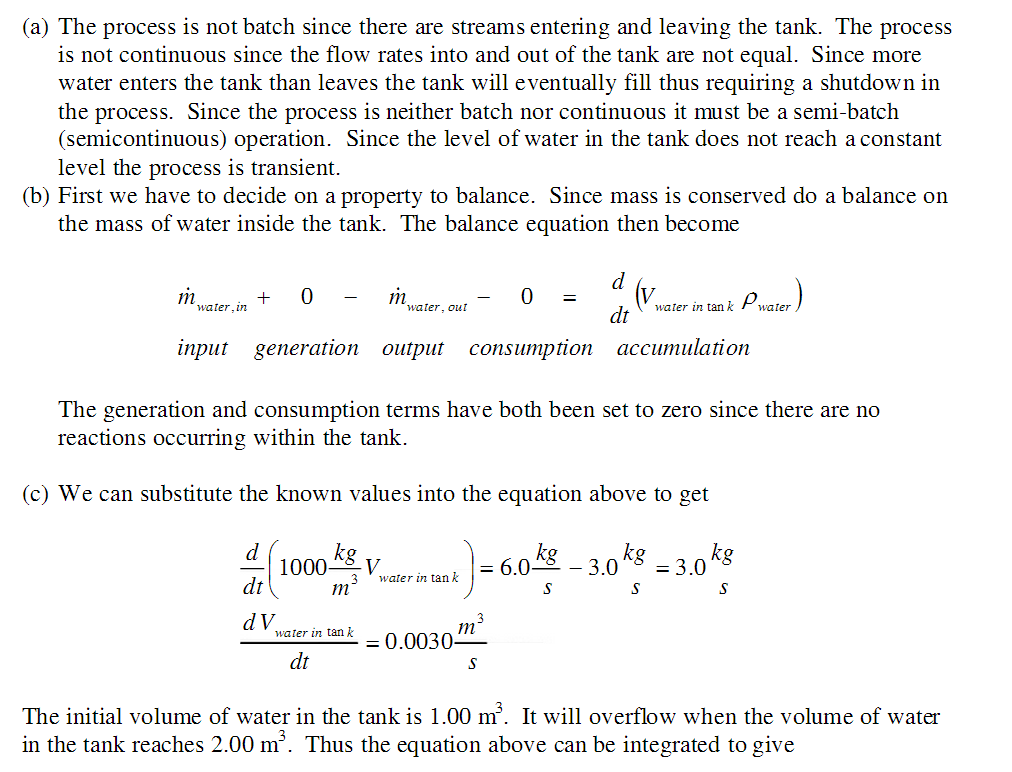# Water (ρ = 1000 kg/m3 ) enters a 2.00 m 3 tank at a rate of 6.00 kg/s and is withdrawn at a rate of 3.00 kg/s. The tank is initially half-full. (a) Is the process continuous, batch, or semi-batch? (b) Is it transient or steady state? (c) Use the general material balance equation to determine how long it will take for the tank to overflow.

Question-AnswerCategory: Material And Energy BalanceWater (ρ = 1000 kg/m3 ) enters a 2.00 m 3 tank at a rate of 6.00 kg/s and is withdrawn at a rate of 3.00 kg/s. The tank is initially half-full. (a) Is the process continuous, batch, or semi-batch? (b) Is it transient or steady state? (c) Use the general material balance equation to determine how long it will take for the tank to overflow.